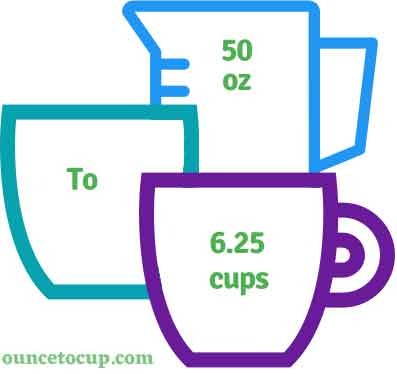# 50 Ounce to Cups (50 oz to cups conversion)

Are you cooking your favorite dish? The detailed chart in the recipe includes the calculation of 50 ounces to cups conversion? Do not worry; check this conversion tool to find how many 50 ounces equal to cups in a minute. This 50 oz to cup converter gives an exact measurement for any recipe you prepare.

Ounce Value:

Ounces

Cup Value:

Cups

50 Ounce = 6.25 Cup
(50 oz = 6.25 cup)

Try our auto 50 ounce to cup calculator (Without Convert Button), Just change the first field value and you got final value.## How many cups is 50 oz?

We know that the volume value of 50 oz is equal to 6.25 cups. If you want to convert 50 fluid oz to an equal number of cups, just divide the volume value by 8. Hence, 50 Ounce is equal to 6.25 cup.

The Answer is: 50 US Fluid Ounces = 6.25 US Cup

50 oz = 6.25 cup

Many of them try to search or find an answer for what is 50 ounces in cups? So, we’ll start with 50 fl oz to cups conversion to know how big is 50 oz.

## How To Calculate 50 fluid oz to cups?

To calculate 50 fluid ounces to an equal number of cups, simply follow the steps below.

Fluid Ounces to Cups formula is:

Cup = Fluid Ounce / 8

Assume that we are finding out how many cups were found in 50 fl oz of water, multiply it either by 0.125 or divide the value by 8 to get the result.

Applying to Formula: Cup = 50 oz / 8 = 6.25 cup.

## How to convert 50 oz to cups?

• To convert 50 fluid ounces to cups,
• Simply multiply the 50 fluid ounces by 0.125, or simply divide them by 8.
• Applying to the formula, cups = 50 ounces * 0.125 [50x0.125] (or) cups = 50 ounces/8 = 50/8 = 6.25.
• Hence, 50 ounces is equal to 6.25 cups.

## Some quick table references for fluid ounce to cup conversions:

Ounce [oz] Cup [c]
1 oz0.125 cup
2 oz0.25 cup
3 oz0.375 cup
4 oz0.5 cup
5 oz0.625 cup
6 oz0.75 cup
7 oz0.875 cup
8 oz1 cup
9 oz1.125 cup
10 oz1.25 cup
11 oz1.375 cup
12 oz1.5 cup

## Reverse Calculation: How many ounces are in 50 cups?

• To convert 50 cups to ounces,
• Simply multiply the 50 cups by 8.
• Then, applying the formula, ounce = 50 cup * 8 [50x8 = 400].
• Hence, 50 cup is equal to 400 ounces.